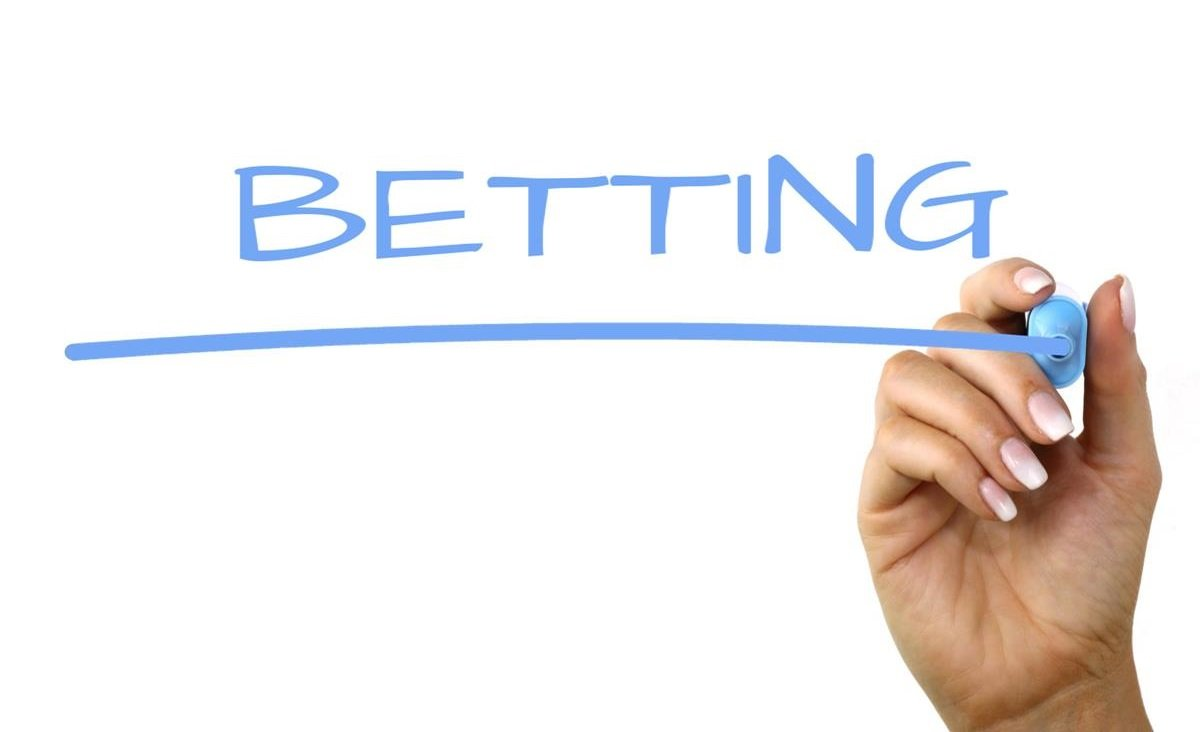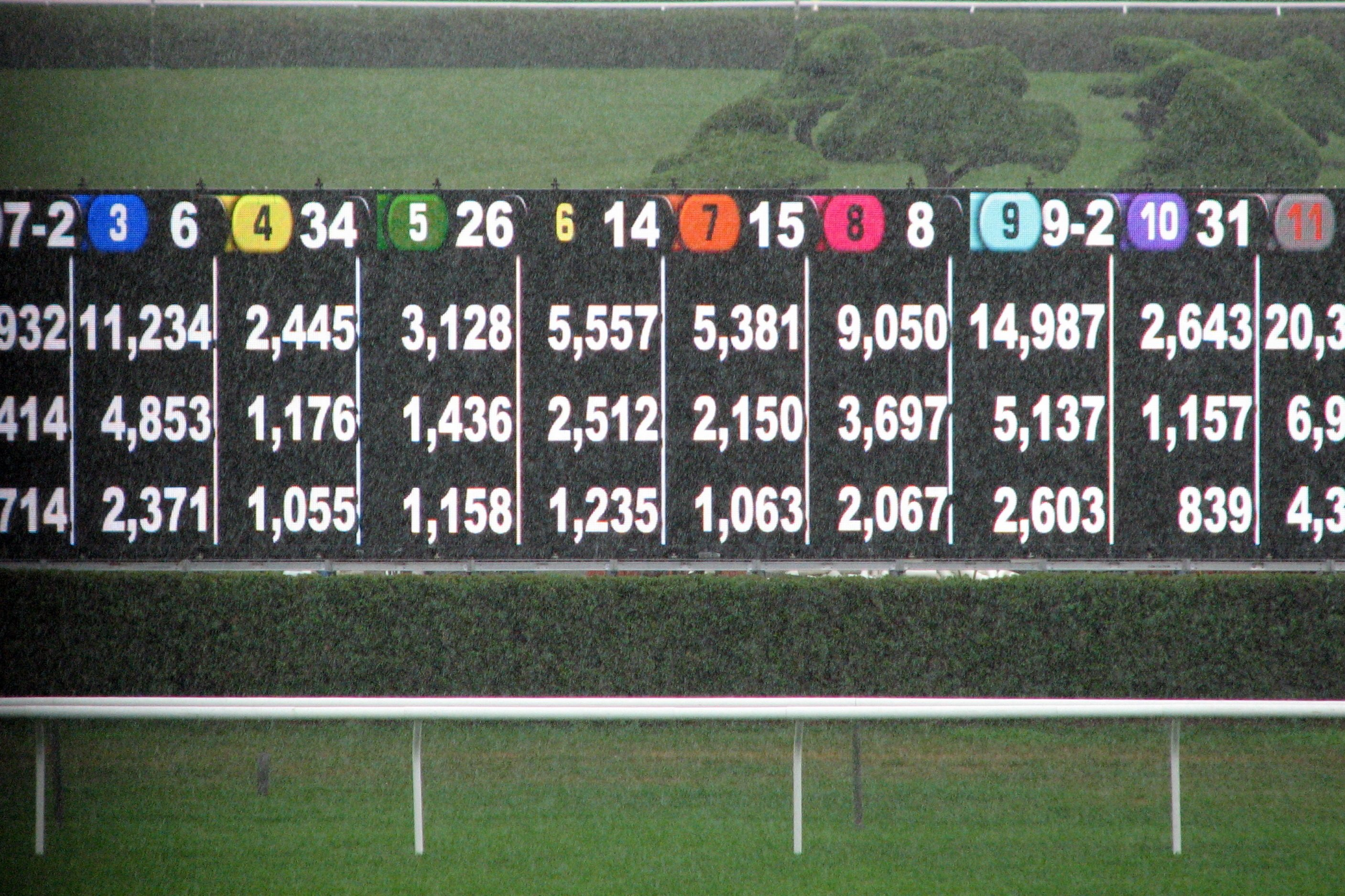Updated: October 2019

# How to Convert Betting Odds into Probability

26 April 2019

Understanding how to convert betting odds into implied probability increases the chances of making a long term profit in the world of sports betting.

It gives you more of an understanding of how a bookmaker thinks an event will turn out and can also enable you to find good value bets, if the implied probability is lower than what you think it should be.

Understanding Betting Odds

First of all, you need to completely understand what betting odds are. These are usually given in one of three ways, which are:

• Decimal (1.80 or 3.00)
• Fractional (5/2 (five to two) or 4/1 (four to one))
• Moneyline/American (+120 or -180)

Regardless of how the odds are shown, they all reflect the possible return from a bet.

The decimal odds represent the return from a single unit of currency. So if the odds are 1.80, you will earn a 0.80 profit from every 1 staked. Staking 100 at odds of 1.80 will return a total payout of 180.

Fractional odds give a fraction such as 5/2 which means for every 2 you stake, you will make 5 profit if the bet is a winner. If you placed a bet of 20 on a 5/2 shot, you would win 50 plus get your 20 stake back.

Moneyline or American odds reflect how much you either need to stake to win 100 (-) or how much you will win for a stake of 100 (+).

Which means, at odds of -180, you would need to stake 180 to win a profit of 100. Odds of +120 means that if you staked 100 and your bet was successful, you would win 120.

Converting Betting Odds into Probability

Now you know what the three types of betting odds are, and how they work, you will be able to convert them into probability using simple equations to give you a percentage. This percentage basically represents the chances of a particular outcome, as believed by the bookmaker.

Convert Decimal Odds into Probability

If we use the decimal odds of 1.80 for this and use the following equation:

• 1 / decimal odds x 100 = implied probability

So, 1 / 1.80 is 0.555 (rounded up to 0.56), giving the mathematical equation of 0.56 x 100 = 56%.

This means that odds of 1.80 reflect a 56% chance of that particular outcome.

To show the formula works every time, we can give another example using odds of 5.00.

1 / 5.00 is 0.2, so 0.2 x 100 = 20%..

Converting Fractional Odds into Probability

Fractional odds are probably the most common form of betting odds, especially in the United Kingdom, and they are also easy to convert into implied probability.

Using odds of 5/2, or five to two, we can work out the chances of that particular outcome.

Firstly, you must add the fraction together by adding the denominator to the numerator. In this case giving a total of 7.

Then, you take the denominator and divide it by the total sum and then multiply it by 100:

• (denominator / 7) x 100 = implied probability.

So, in this case it’s (2 / 7) x 100 = 28.57%

Converting Moneyline/American Odds into Probability

Converting Moneyline, or American odds into implied probability is a little more complicated, as it requires different equations depending on whether the odds are plus or minus.

We will start with the equation for minus moneyline odds. However, you must use the odds without the minus symbol in front. So, the equation is:

• ((minus moneyline odds) / (minus moneyline odds +100)) x 100 = implied probability

So, using odds of -180 we would get the equation of:

• (180 / 280) x 100 = ?

Which works out at a total implied probability of 64.3%

For plus betting odds, the equation changes to

• (100 / (plus moneyline odds +100)) x 100

So, if we used odds of +120, we would get an equation looking like the one below.

This is, (100 / 220) x 100 = implied probability.

The implied probability from this equation is 45.45%.

Using These Conversions to Make a Profit

Obviously, just because someone thinks something is going to happen doesn’t necessarily mean it will. But, being armed with the knowledge of how to convert betting odds into implied probability enables you to work out your best betting options.

You believe, for example, a football player has a 70% chance of scoring during a match but the betting odds convert into implied probability of 56%. This means you believe it will happen more than the bookmaker does, which means you will get better value odds than you think you should.

Finding value is just one reason that being able to convert odds into implied probability is an extremely important thing to know.

Other Guide22 July 2019

Most Common Betting Mistakes – Guide for Beginners

In sports betting there are many betting terms which are not easy to understand for a person who has never bet before. It’s very complex ...

Read More19 July 2019

What is Point Spread Betting – Guide for Beginners

In sports betting there are many betting terms which are not easy to understand for a person who has never bet before. It’s very complex ...

Read More19 July 2019

Value Odds – Guide for Beginners

In sports betting there are many betting terms which are not easy to understand for a person who has never bet before. It’s very complex ...

Read More